# DBSCAN clustering algorithm in Python (with example dataset)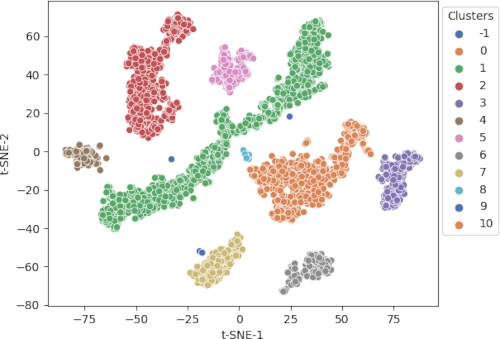## What is DBSCAN?

Density Based Spatial Clustering of Applications with Noise (abbreviated as DBSCAN) is a density-based unsupervised clustering algorithm. In DBSCAN, clusters are formed from dense regions and separated by regions of no or low densities.

DBSCAN computes nearest neighbor graphs and creates arbitrary-shaped clusters in datasets (which may contain noise or outliers) as opposed to k-means clustering, which typically generates spherical-shaped clusters

Unlike k-means clustering, DBSCAN does not require specifying the number of clusters initially. However, DBSCAN requires two parameters viz. the radius of neighborhoods for a given data point p (`eps` or `ε`) and the minimum number of data points in a given `ε`-neighborhood to form clusters (`minPts`).

DBSCAN is also useful for clustering non-linear datasets.

## DBSCAN common terminologies

Here we will discuss the core point, border point, noise point (Outlier), and density reachable terminologies used in DBSCAN.

The randomly selected data point p is called a core point if there are more than a minimum number of points (`minPts`) within a `ε`-neighborhood of p.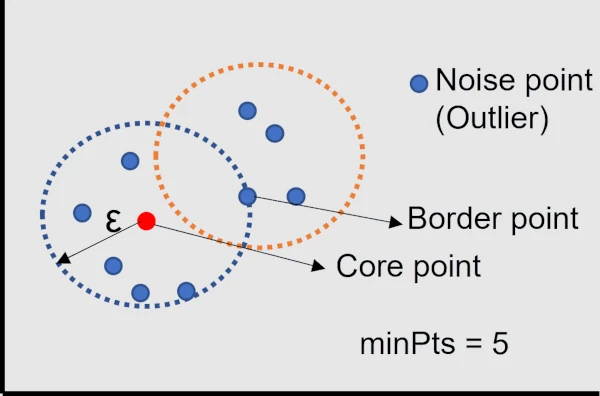A data point is called a border point if it is within a `ε`-neighborhood of p and it has fewer than the minimum number of points (`minPts`) within its `ε`-neighborhood.

If a point that is not a core point or border point is called a Noise point (Outlier).

A point x is directly density reachable from point p if a point p is a core point and x is in p’s `ε`-neighborhood. A point y is density reachable from point p if a point y is directly density reachable to core point x, which is also density reachable to core point p.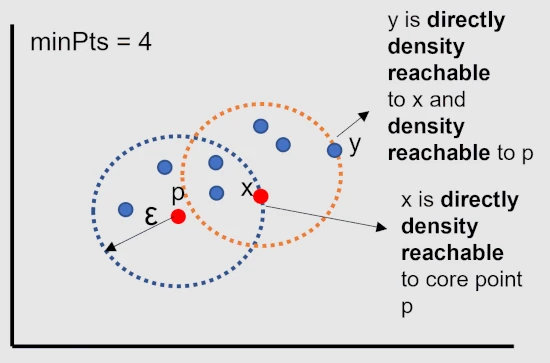All points within a `ε`-neighborhood of a core point belongs to same clusters and all points in a cluster are directly density reachable to core point.

The points which are not density reachable from any core points are called noise (outliers) points.

## Steps involved in DBSCAN clustering algorithm

1. Choose any point p randomly
2. Identify all density reachable points from p with `ε` and `minPts` parameter
3. If p is a core point, create a cluster (with `ε` and `minPts`)
4. If p is a border point, visit the next point in a dataset
5. Continue the algorithm until all points are visited

## Perform DBSCAN clustering in Python

To perform DBSCAN clustering in Python, you will require to install `sklearn`, `pandas`, and `matplotlib` Python packages. Check for how to install Python packages

### Get dataset

For clustering using DBSCAN, I am using a single-cell gene expression dataset of Arabidopsis thaliana root cells processed by a 10x genomics Cell Ranger pipeline. The dataset is preprocessed t-SNE dimensionality reduction technique. Now, I will use the t-SNE embedding vectors to identify the clusters using DBSCAN.

``````import pandas as pd

t-SNE-1    t-SNE-2
0  10.846841 -16.712580
1  24.794334 -16.775398

# check the shape of dataset
print(df.shape)
(4406, 2)
``````

This dataset has 4406 rows and two features. This is unlabelled dataset (no cluster information). I will identify the cluster information on this dataset using DBSCAN.

### Compute required parameters for DBSCAN clustering

DBSCAN requires `ε` and `minPts` parameters for clustering. The `minPts` parameter is easy to set. The `minPts` should be 4 for two-dimensional dataset. For multidimensional dataset, `minPts` should be `2 * number of dimensions`. For example, if your dataset has 6 features, set `minPts = 12`. Sometimes, domain expertise is also required to set a `minPts` parameter.

Another question is what optimal value should be used for the `ε` parameter. The `ε` parameter is difficult to set and depends on the distance function. Sometimes, domain expertise is also required to set a `ε` parameter. The `ε` should be as small as possible.

To determine the optimal `ε` parameter, I will compute the k-nearest neighbor (kNN) distances (average distance of every data point to its k-nearest neighbors) of an input dataset using the k-nearest neighbor method (unsupervised nearest neighbors learning). For finding the k-nearest neighbor, I will use the `sklearn.neighbors.NearestNeighbors` function.

`NearestNeighbors` function requires `n_neighbors` (number of neighbors) parameter, which can be same as the `minPts` value.

``````import numpy as np
from sklearn.neighbors import NearestNeighbors
# n_neighbors = 5 as kneighbors function returns distance of point to itself (i.e. first column will be zeros)
nbrs = NearestNeighbors(n_neighbors = 5).fit(df)
# Find the k-neighbors of a point
neigh_dist, neigh_ind = nbrs.kneighbors(df)
# sort the neighbor distances (lengths to points) in ascending order
# axis = 0 represents sort along first axis i.e. sort along row
sort_neigh_dist = np.sort(neigh_dist, axis = 0)
``````

Now, get the sorted kth column (distances with kth neighbors) and plot the kNN distance plot,

``````import matplotlib.pyplot as plt
k_dist = sort_neigh_dist[:, 4]
plt.plot(k_dist)
plt.ylabel("k-NN distance")
plt.xlabel("Sorted observations (4th NN)")
plt.show()
``````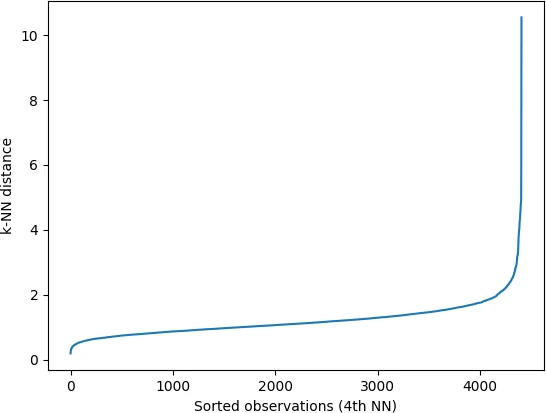In the k-NN distance plot, you should look for the “knee” or “elbow” point (a threshold value where you see a sharp change) of the curve to find the optimal value of `ε`.

Identifying the exact knee point could be difficult visually. In the below plot, the knee point can occur at any point between 2 to 5 i.e. the points below knee point belong to a cluster, and points above the knee point are noise or outliers (noise points will have higher kNN distance). You should run DBSCAN based on different values of `ε` (between 2 and 5) to find the best `ε` that gives the best clustering.

Additionally, to get an estimate of knee point, you can use the `KneeLocator()` function from the `kneed` package.

``````from kneed import KneeLocator
kneedle = KneeLocator(x = range(1, len(neigh_dist)+1), y = k_dist, S = 1.0,
curve = "concave", direction = "increasing", online=True)

# get the estimate of knee point
print(kneedle.knee_y)
4.5445133515748894
``````

Distance plot,

``````kneedle.plot_knee()
plt.show()
``````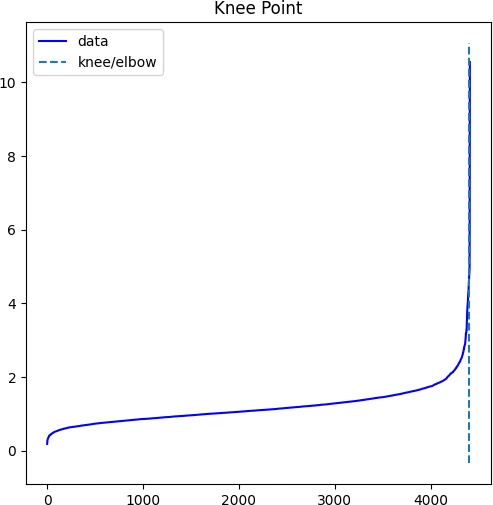We will use 4.54 as optimum value of `ε` for DBSCAN clustering

### Compute DBSCAN clustering

Now, we have calculated `ε` and `minPts` parameters for DBSCAN clustering. We will pass these parameters to the DBSCAN to predict the clusters using the `sklearn.cluster.DBSCAN` class.

``````from sklearn.cluster import DBSCAN
clusters = DBSCAN(eps = 4.54, min_samples = 4).fit(df)
# get cluster labels
clusters.labels_
# output
array([0, 0, 1, ..., 1, 1, 1], dtype=int64)

# check unique clusters
set(clusters.labels_)
{0, 1, 2, 3, 4, 5, 6, 7, 8, 9, 10, -1}
# -1 value represents noisy points could not assigned to any cluster
``````

Choosing appropriate DBSCAN parameters can be harder sometimes. If you select `ε` based on your domain expertise, you should play with different values of `minPts` to get better insights from clustering.

Get each cluster size,

``````from collections import Counter
Counter(clusters.labels_)
# output
Counter({1: 1524, 0: 870, 2: 769, 3: 301, 7: 283, 5: 246, 6: 232, 4: 153, 8: 11, 10: 8, 9: 6, -1: 3})
``````

DBSCAN clustering identified 11 clusters with given parameters. The largest cluster (1) has 1524 data points and the smallest cluster (9) has 6 data points.

### Visualization of DBSCAN clustering

Visualize the cluster as a scatter plot and color the clusters using predicted class labels,

``````import seaborn as sns
import matplotlib.pyplot as plt
p = sns.scatterplot(data = df, x = "t-SNE-1", y = "t-SNE-2", hue = clusters.labels_, legend = "full", palette = "deep")
sns.move_legend(p, "upper right", bbox_to_anchor = (1.17, 1.), title = 'Clusters')
plt.show()
``````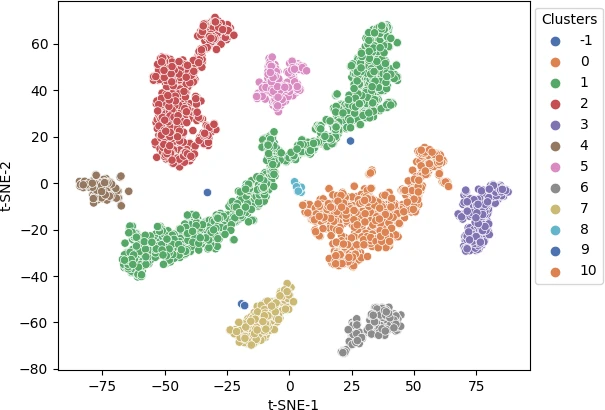Different colors represent different predicted clusters. Blue represents noisy points (-1 cluster).

### DBSCAN limitations

• DBSCAN is computationally expensive (less scalable) and more complicated clustering method as compared to simple k-means clustering
• DBSCAN is sensitive to input parameters, and it is hard to set accurate input parameters
• DBSCAN depends on a single value of `ε` for all clusters, and therefore, clusters with variable densities may not be correctly identified by DBSCAN
• DBSCAN is a time-consuming algorithm for clustering

## Enhance your skills with courses on machine learning

If you have any questions, comments, corrections, or recommendations, please email me at reneshbe@gmail.com